Question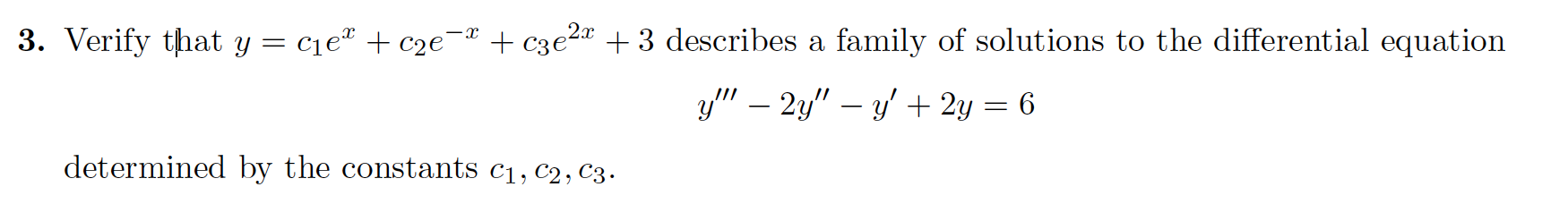We need at least 10 more requests to produce the answer.

0 / 10 have requested this problem solution

The more requests, the faster the answer.

All students who have requested the answer will be notified once they are available.

#### Earn Coins

Coins can be redeemed for fabulous gifts.

Similar Homework Help Questions
• ### In this problem, y = Ciex + c2e-X is a two-parameter family of solutions of the...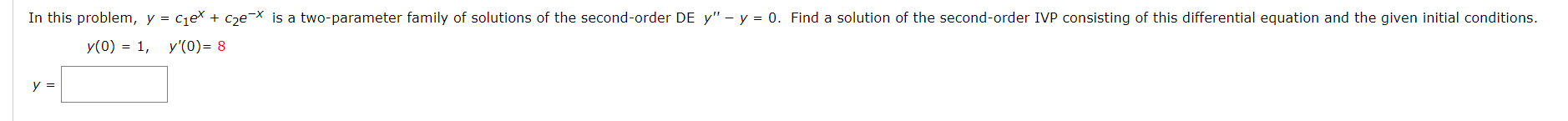In this problem, y = Ciex + c2e-X is a two-parameter family of solutions of the second-order DE y" - y = 0. Find a solution of the second-order IVP consisting of this differential equation and the given initial conditions. y(0) = 1, y'O)= 8

• ### Verify that the given function is a solution to the given differential equation (c1 and c2...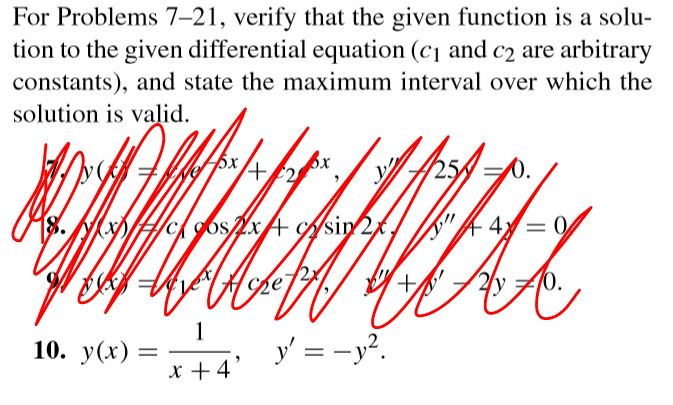Verify that the given function is a solution to the given differential equation (c1 and c2 are arbitrary constants) and state the maximum interval over which the solution is valid. For Problems 7-21, verify that the given function is a solu- tion to the given differential equation (cy and c2 are arbitrary constants), and state the maximum interval over which the solution is valid. ya Sx +42 25 WID#cigos x A Asin 2%, = 0 BAWK vel Hope 2y +10....

• ### Chapter 4, Section 4.4, Additional Question 01 Use the method of variation of parameters to determine the general solution of the given differential equation. y4 +2y y 11sin (t) Use C1, C2, C3, f...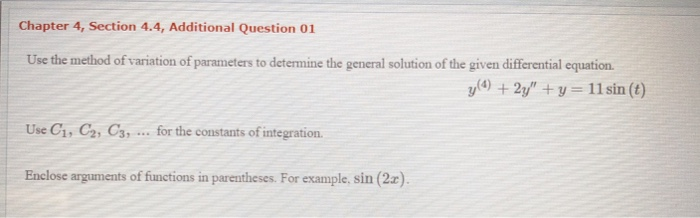Chapter 4, Section 4.4, Additional Question 01 Use the method of variation of parameters to determine the general solution of the given differential equation. y4 +2y y 11sin (t) Use C1, C2, C3, for the constants of integration. Enclose arguments of functions in parentheses. For example, sin (2x) Chapter 4, Section 4.4, Additional Question 01 Use the method of variation of parameters to determine the general solution of the given differential equation. y4 +2y y 11sin (t) Use C1, C2,...

• ### Use the method of variation of parameters to determine the general solution of the given differential...

Use the method of variation of parameters to determine the general solution of the given differential equation. y′′′−2y′′−y′+2y=e6t Use C1, C2, C3, ... for the constants of integration.

• ### Use the method of variation of parameters to determine the general solution of the given differential equation. y(4)+2y′′+y=3sin(t) Use C1, C2, C3, ... for the constants of integration. Enclose argume...

Use the method of variation of parameters to determine the general solution of the given differential equation. y(4)+2y′′+y=3sin(t) Use C1, C2, C3, ... for the constants of integration. Enclose arguments of functions in parentheses. For example, sin(2x). y(t)=

• ### Verify that the given function is a solution to the given differential equation (c1 and c2...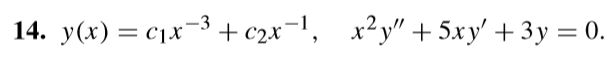Verify that the given function is a solution to the given differential equation (c1 and c2 are arbitrary constants), and state the maximum interval over which the solution is valid. 14. y(x) = cix-3 + c2x-1, x2y" + 5xy' + 3y = 0.

• ### verify that the given function is a solution to the given differential equation (c1 andc2 arbitrary...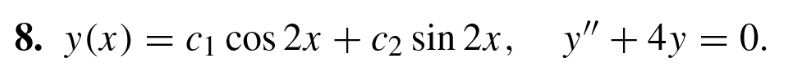verify that the given function is a solution to the given differential equation (c1 andc2 arbitrary constants), and state the maximum interval over which the solution is valid. 8. y(x) = cj cos 2x + c2 sin 2x, y + 4y = 0.

• ### Solve the differential equation. dy dx 3.c2e- y=In(x3 + C) y=Cln(x) y=+*+ Oy=ln(3x + C)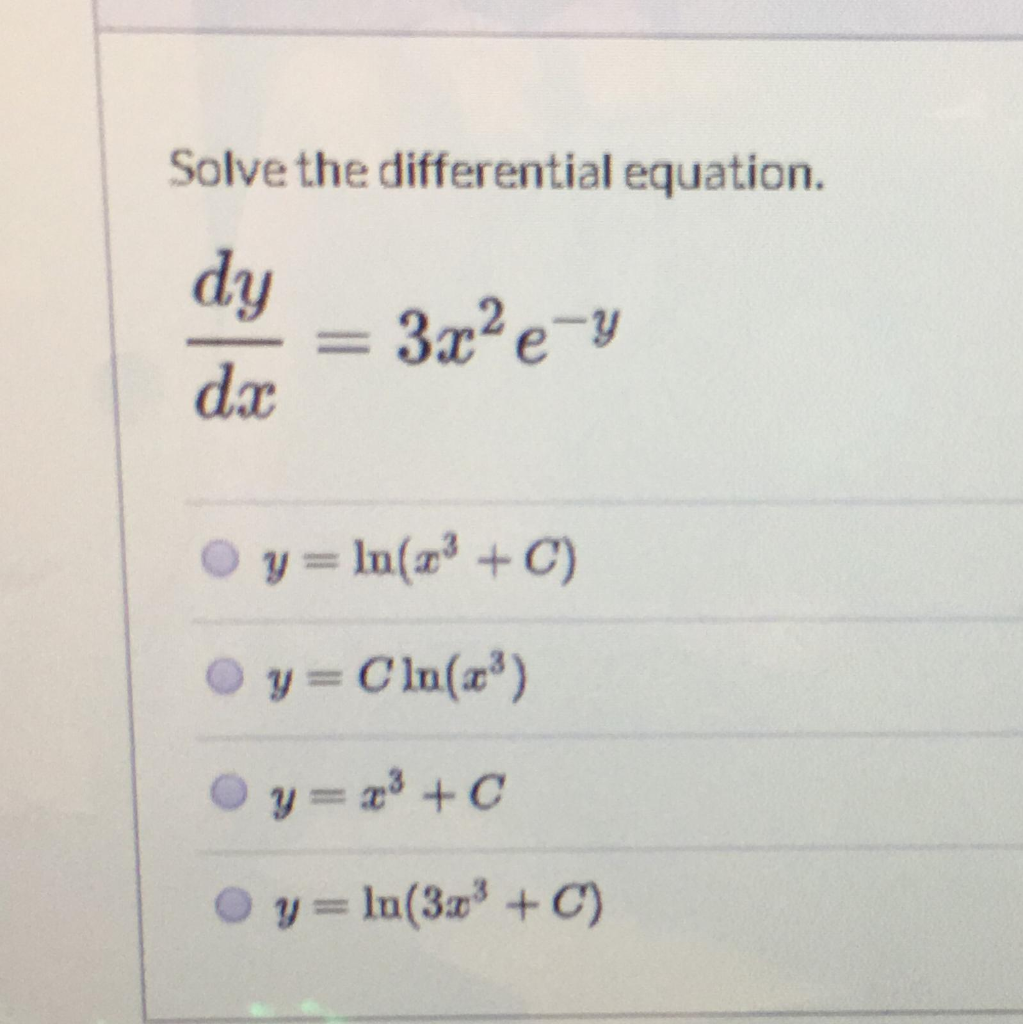Solve the differential equation. dy dx 3.c2e- y=In(x3 + C) y=Cln(x) y=+*+ Oy=ln(3x + C)

• ### (a) Verify that all members of the family y (5)1/2 (c- x2)-1/2 are solutions of the differential equationy y (b) Find a solution of the initial-value problem (a) Verify that all members of th...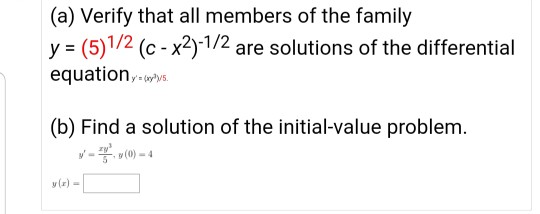(a) Verify that all members of the family y (5)1/2 (c- x2)-1/2 are solutions of the differential equationy y (b) Find a solution of the initial-value problem (a) Verify that all members of the family y (5)1/2 (c- x2)-1/2 are solutions of the differential equationy y (b) Find a solution of the initial-value problem

• ### (3 points) (a) Find the general solution to y′′+2y′=0. Give your answer as y=... . In...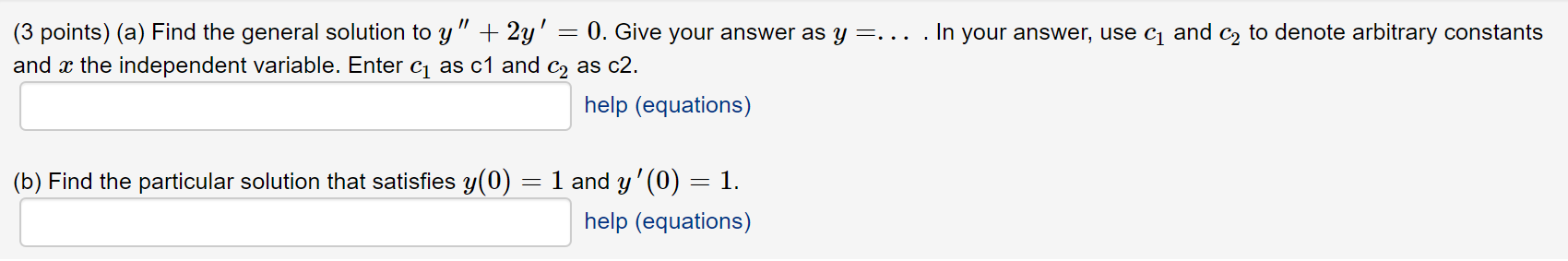(3 points) (a) Find the general solution to y′′+2y′=0. Give your answer as y=... . In your answer, use c1 and c2 to denote arbitrary constants and x the independent variable. Enter c1 as c1 and c2 as c2. (3 points) (a) Find the general solution to y" + 2y' = 0. Give your answer as y=... . In your answer, use c1 and c2 to denote arbitrary constants and x the independent variable. Enter cı as c1 and C2...